## Cubes and Dices

#### Cubes and Dices

1. Two positions of a dice are shown below. When 4 is at the top what number will be at the bottom?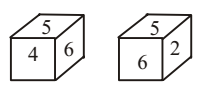1. On the basis of following pattern in given dices , we can say that
The numbers 2, 4 and 5 cannot be on the opposite face of 6.

##### Correct Option: C

On the basis of following pattern in given dices , we can say that
The numbers 2, 4 and 5 cannot be on the opposite face of 6.
From the two views of dice, it is clear that 2 is at bottom when 4 is at top.

1. Observe the faces of the dice and then answer the question.Which number is on the face opposite to 4?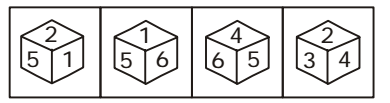1. As per the given pattern in question figure , we can find the similar next position of given dice .
The numbers 1, 2, 4 and 6 are on the adjacent faces of number 5.
Therefore, number 3 will lie opposite 5.
The numbers 2, 5 and 6 are on the adjacent faces of number 1.

##### Correct Option: A

As per the given pattern in question figure , we can find the similar next position of given dice .
The numbers 1, 2, 4 and 6 are on the adjacent faces of number 5.
Therefore, number 3 will lie opposite 5.
The numbers 2, 5 and 6 are on the adjacent faces of number 1.
Therefore, 4 lies opposite 1.

1. Four positions of a dice are given below. Identify the number at the bottom when top is 6.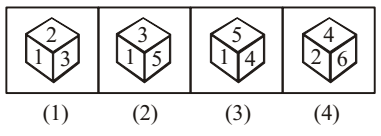1. On the basis of following pattern in given dices , we can say that
The numbers 2, 3, 4 and 5 cannot be on the opposite faces of the number 1.

##### Correct Option: B

On the basis of following pattern in given dices , we can say that
The numbers 2, 3, 4 and 5 cannot be on the opposite faces of the number 1.
Therefore 1 lies opposite to 6.

1. Three positions of a dice are given below. Identify the number on the face opposite to 6.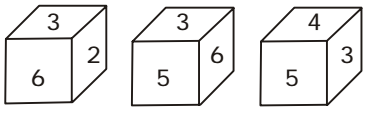1. On the basis of following pattern in given dices , we can say that
The numbers 2, 4, 5 and 6 cannot be on the face opposite to 3.
The numbers 1, 3, 4 and 6 cannot be on the face opposite to 5.

##### Correct Option: B

On the basis of following pattern in given dices , we can say that
The numbers 2, 4, 5 and 6 cannot be on the face opposite to 3.
The numbers 1, 3, 4 and 6 cannot be on the face opposite to 5.
Therefore, 2 lies opposite 5.
Clearly, 4 lies opposite 6.

1. Study the following figures and find out the number opposite to 3.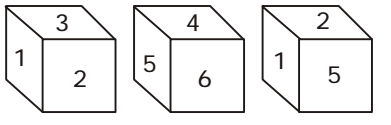1. On the basis of following pattern in given dices , we can say that
The numbers 1, 2, 4 and 6 are on adjacent faces of the number 5.

##### Correct Option: C

On the basis of following pattern in given dices , we can say that
The numbers 1, 2, 4 and 6 are on adjacent faces of the number 5.
Therefore, the number 3 lies opposite to 5.